Practice Test: Mechanical Engineering (ME)- 2

# Practice Test: Mechanical Engineering (ME)- 2

Test Description

## 65 Questions MCQ Test GATE Mechanical (ME) 2023 Mock Test Series | Practice Test: Mechanical Engineering (ME)- 2

Practice Test: Mechanical Engineering (ME)- 2 for Mechanical Engineering 2023 is part of GATE Mechanical (ME) 2023 Mock Test Series preparation. The Practice Test: Mechanical Engineering (ME)- 2 questions and answers have been prepared according to the Mechanical Engineering exam syllabus.The Practice Test: Mechanical Engineering (ME)- 2 MCQs are made for Mechanical Engineering 2023 Exam. Find important definitions, questions, notes, meanings, examples, exercises, MCQs and online tests for Practice Test: Mechanical Engineering (ME)- 2 below.
Solutions of Practice Test: Mechanical Engineering (ME)- 2 questions in English are available as part of our GATE Mechanical (ME) 2023 Mock Test Series for Mechanical Engineering & Practice Test: Mechanical Engineering (ME)- 2 solutions in Hindi for GATE Mechanical (ME) 2023 Mock Test Series course. Download more important topics, notes, lectures and mock test series for Mechanical Engineering Exam by signing up for free. Attempt Practice Test: Mechanical Engineering (ME)- 2 | 65 questions in 180 minutes | Mock test for Mechanical Engineering preparation | Free important questions MCQ to study GATE Mechanical (ME) 2023 Mock Test Series for Mechanical Engineering Exam | Download free PDF with solutions
 1 Crore+ students have signed up on EduRev. Have you?
Practice Test: Mechanical Engineering (ME)- 2 - Question 1

### There are two lines made by joining points A, B,C. B lies between the line joining A and C. Is the distance between the A and C passes through the B more then 7 Km. (i) The distance between A and B is 6 Km (ii) Distance between B to C is 2 Km long,

Detailed Solution for Practice Test: Mechanical Engineering (ME)- 2 - Question 1

From Statement I

We know the distance between Points B and C but we do not know about the distance between A and B

Statement II

This statement tells us about the distance between A and B and by using Both statement we can calculate the distance between A and C that is longer than the 2 Km.

Practice Test: Mechanical Engineering (ME)- 2 - Question 2

### Direction: In the given question, a word/phrase is given followed by three statements; I, II and III. Choose the pair of sentences which can be combined using the given word/ phrase when used at the beginning of the new sentence.AndI: When Lionel Messi, Ronaldo and Neymar kick and dribble the football, they don’t remain confined to Argentina, Portugal or Brazil, respectively.II: Let the entire world realise that through sports, especially football, we all can cement our bonds, wash away our bitterness and prejudices.III: Live with a greater sense of closeness and joy with each other.

Detailed Solution for Practice Test: Mechanical Engineering (ME)- 2 - Question 2 ‘And’ is used as a conjunction here which is used to introduce an additional comment or interjection.

Here sentences II and III are displaying same sense that is realizing the benefit of football in our lives whereas sentence I is providing entirely different aspect of football as a sport.

Hence option (B) will be the most appropriate choice.

New sentence: Let the entire world realise that through sports, especially football, we all can cement our bonds, wash away our bitterness and prejudices, and live with a greater sense of closeness and joy with each other.

Practice Test: Mechanical Engineering (ME)- 2 - Question 3

### Which of the following is the MOST SIMILAR in meaning to Accreditation?

Detailed Solution for Practice Test: Mechanical Engineering (ME)- 2 - Question 3 Accreditation = an acknowledgement of a person's responsibility for or achievement of something.

Certification = an official document attesting to a status or level of achievement.

Meticulous = showing great attention to detail; very careful and precise.

Lurid = very vividly shocking.

Suppressive = tending or acting to suppress.

Agreement = harmony or accordance in opinion or feeling.

Practice Test: Mechanical Engineering (ME)- 2 - Question 4

In how many ways can you place 2 white bishops on an empty chess board?

Detailed Solution for Practice Test: Mechanical Engineering (ME)- 2 - Question 4 One white bishop can be placed on any of the 32 white boxes and the other white bishop can be placed on any of the 32 black boxes.

Ways of doing that = 32C1 × 32C1

= 32 × 32 = 1024

Practice Test: Mechanical Engineering (ME)- 2 - Question 5

Direction: In the question below are given statements followed by some conclusions. You have to take the given statements to be true even if they seem to be at variance with commonly known facts. Read all the conclusions and then decide which of the given conclusions logically follows from the given statements disregarding commonly known facts.

Statement:

No physics is maths.

Some chemistry is maths.

All sciences are chemistry.

Conclusion:

I. No science is physics.

II. Some physics are science.

III. Some physics are chemistry.

Detailed Solution for Practice Test: Mechanical Engineering (ME)- 2 - Question 5 The least possible Venn diagram for the given statements is as follows.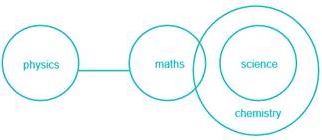Conclusions:

I. No science is physics → False (It is possible but not definite).

II. Some physics are science → False (It is possible but not definite)

III. Some physics are chemistry → False (It is possible but not definite)

Conclusion I and II form complementary pair.

Hence, either conclusion I or II follows.

Practice Test: Mechanical Engineering (ME)- 2 - Question 6

Direction: Read the information carefully and give the answer of the following questions-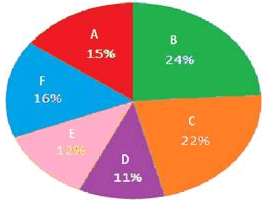(This pie chart shows the percentage of students appear in six different exams in 2016)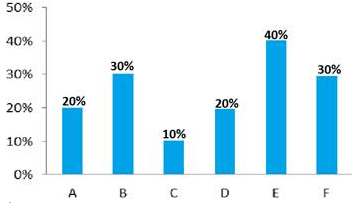(This bar graph shows the percentage of failed students which appear in these six different exams in 2016)

If in 2016, total number of failed students in exam F was 4080, then how many passed students appears in the exam B?

Detailed Solution for Practice Test: Mechanical Engineering (ME)- 2 - Question 6

14280 ( Let total number of students be = 100z.

Total number of students appears in exam F = 16% of 100z = 16z;

Total number of failed students in exam F = 30% of 16z = 4.8z = 4080;

=> z = 850;

Total number of passed students appears in the exam B = (100 – 30)% of 24% of 100z = 16.8z

= 16.8 × 850 = 14280 ;)

Practice Test: Mechanical Engineering (ME)- 2 - Question 7

Direction: Two sentences with two blanks in each, followed by five alternatives with two words in each, are given. Choose that option as the answer which can fill both the blanks of both the sentences.

i. A sinking feeling of panic ________ over them and a temporary paralyzing fear engulfed them ________.

ii. Being a cleanliness freak, she ________ the floor and went down to the market only after the house was _________ clean.

Detailed Solution for Practice Test: Mechanical Engineering (ME)- 2 - Question 7 ‘Sweep’ is to clean (an area) by brushing away dirt or litter. It fits the first blank of the second sentence, as the subject is cleaning the floor. Further, ‘sweep over’ means to overcome or overwhelm, thus fits in the first blank of the first sentence. The second blank of both the sentences can be filled by "completely", thus conveying an appropriate sense. Thus, option D is the correct answer.
Practice Test: Mechanical Engineering (ME)- 2 - Question 8

In the following question, some statements followed by some conclusions are given. Taking the given statements to be true even if they seem to be at variance from commonly known facts, read all the conclusions and then decide which of the given conclusions logically follows the given statements.

Statement:

Tension is detrimental to physical and mental health.

Conclusions:

I. To be healthy one should be free from tension.

II. Mental health depends upon the tension one experiences.

Detailed Solution for Practice Test: Mechanical Engineering (ME)- 2 - Question 8 Conclusion I. To be healthy one should be free from tension. (Follows as both physical and mental health are affected from tension.)

Conclusion II. Mental health depends upon the tension one experiences. (Follows).

Hence, option B is correct.

Practice Test: Mechanical Engineering (ME)- 2 - Question 9

4 identical solid spheres are melted and reformed into a solid hemisphere. Then, the ratio of the curved surface area of the hemisphere to half of the surface area of a single sphere is -

Detailed Solution for Practice Test: Mechanical Engineering (ME)- 2 - Question 9 Let radius of sphere be ‘r’ and radius of hemisphere be ‘R’.

Then, ATQ, Volume of Spheres = Volume of Hemisphere

4 x (4/3)πr3 = (2/3)πR3

Or, R / r = (8)1/3 = 2 …(1)

C.S.A of hemisphere = 2πR2

And, Surface area of sphere = 4πr2

ATQ, 2πR2 :( ½) of 4πr2

= 2πR2 :2πr2 = R2 :r2 {using (1)} ×

= 4 :1

Practice Test: Mechanical Engineering (ME)- 2 - Question 10

A study of people who reduced the calories they consumed has found the strongest evidence yet that such restrictions slow down metabolism, raising hopes that a low calories lifestyle or treatments stimulating biological effects of restricted eating, could prolong health in old age. The report provides the most robust evidence to date that everything we have learnt in other animals can be applied to humans.

Which of the following argument will prove that the above conclusion is flawed?

Detailed Solution for Practice Test: Mechanical Engineering (ME)- 2 - Question 10 The given passage gives the benefits of reducing calories to attain a healthier lifestyle and out of the given options, option B seems to give a contradictory fact stating that the lack of required calories may cause problems to the body. Hence, option B is correct.
Practice Test: Mechanical Engineering (ME)- 2 - Question 11

If two gears have moment of inertia as I1 and I2 respectively and mesh with a speed ratio ω21 = n then equivalent moment of inertia of both gears referred to first one is?

Detailed Solution for Practice Test: Mechanical Engineering (ME)- 2 - Question 11 between shaft 1 and shaft 2 the kinetic energy will be same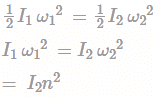So equivalent moment of inertia w.r.t to first one = I1 + n2I2

Practice Test: Mechanical Engineering (ME)- 2 - Question 12

A solid body formed by joining the base of a right circular cone of height equals to 150mm to the equal base of a right circular cylinder of height h = 45mm. Calculate the distance of the centre of mass of the solid from its plane face?

Detailed Solution for Practice Test: Mechanical Engineering (ME)- 2 - Question 12 As the body is symmetrical about the vertical axis, therefore its centre of gravity will lie on this axis as shown in Fig. Let r be the radius of the cylinder base in cm. Now let base of the cylinder be the axis of reference.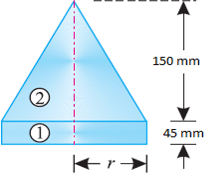(i). Cylinder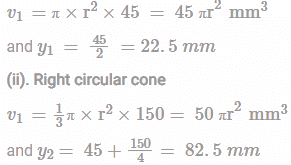We know that distance between centre of gravity of the section and base of the cylinder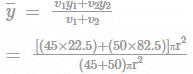= 54.08 mm

Practice Test: Mechanical Engineering (ME)- 2 - Question 13

Two steel sheet of 1 mm thick are resistance welded in a lap joint with a current of 10000 A for 0.1 sec. The effective resistance of joint can be taken as 100μΩ. The joint can be considered as cylinder φ5 of mm and 1.5 mm height. Ρsteel = 0.00786 g/mm3. The heat lost surroundings if the heat required to melt steel is 10 J/mm3 is?

Detailed Solution for Practice Test: Mechanical Engineering (ME)- 2 - Question 13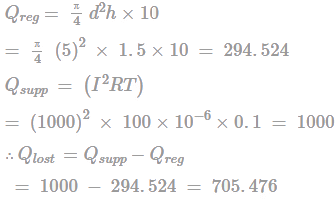Practice Test: Mechanical Engineering (ME)- 2 - Question 14

A plate 1 mm distance from a fixed plate, is moving at 500 mm/s by a force induces a shear stress of 0.3 kg(f)/m2. The kinematic viscosity of the fluid (mass density 1000 kg/m3) flowing between two plates (in Stokes) is?

Detailed Solution for Practice Test: Mechanical Engineering (ME)- 2 - Question 14 Given, y = 0.001 m, v = 0.5 m/s,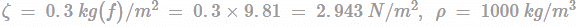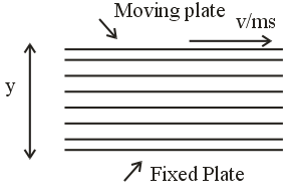Using Newton’ law of viscosity,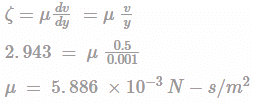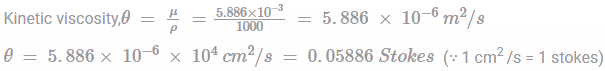Practice Test: Mechanical Engineering (ME)- 2 - Question 15

For a circular shaft of diameter d subjected to torque T, the maximum value of the shear stress is?

Detailed Solution for Practice Test: Mechanical Engineering (ME)- 2 - Question 15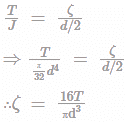Practice Test: Mechanical Engineering (ME)- 2 - Question 16

Figure shows the cross-section of a cast iron beam. Determine the moments of inertia of the section about horizontal axis passing through the centroid of the section.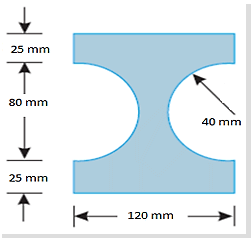Detailed Solution for Practice Test: Mechanical Engineering (ME)- 2 - Question 16 Given fig. is symmetrical about X axis and Y axis therefore its center of gravity will lie at the center of the rectangle.

Moment of inertia of the rectangular section about its horizontal axis passing through its centre of gravity, = bd3/12 = 120 x (130/2) = 21.97mm4 and moment of inertia of the circular section about a horizontal axis passing through its centre of gravity,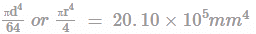Moment of inertia of the whole section about horizontal axis passing through the centroid of the section,

IXX = (21.97 × 106) – (2.010 × 106) = 19.97 × 106 mm4

Practice Test: Mechanical Engineering (ME)- 2 - Question 17

A liquid with specific gravity of 0.94 undergoes a reduction in volume of 0.22% when subjected to an increase in pressure of 1400kPa. The velocity of propagation of sound in this liquid will be ___________m/s

Detailed Solution for Practice Test: Mechanical Engineering (ME)- 2 - Question 17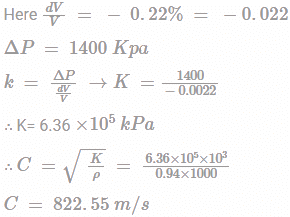Practice Test: Mechanical Engineering (ME)- 2 - Question 18

Heat flows through a composite slab as shown below, The depth of the slab is 10 cm. The k values are in W/m-K. The heat flow rate through the composite wall in W is?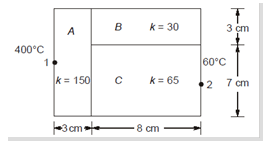Detailed Solution for Practice Test: Mechanical Engineering (ME)- 2 - Question 18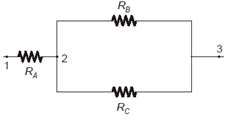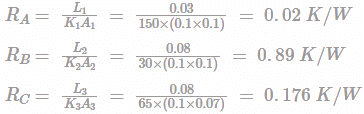Equivalent resistance between B and C-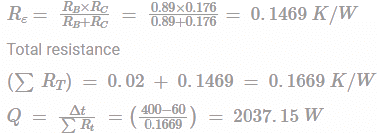Practice Test: Mechanical Engineering (ME)- 2 - Question 19

A matrix has eigen values of ‒ 1 and ‒ 2 respectively. The corresponding eigen vectors are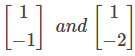respectively. The matrix is?

Detailed Solution for Practice Test: Mechanical Engineering (ME)- 2 - Question 19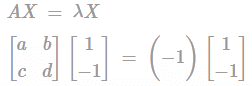a -b = -1....(i)

c - d = 1.....(ii)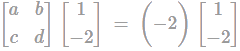⇒ a - 2b = -2...(iii)

c - 2d = 4.....(iV)

From equation (i) and (iii), a = 0 and b = 1

From equation (ii) and (iv), c = - 2 and d = - 3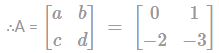Practice Test: Mechanical Engineering (ME)- 2 - Question 20

The turing moment diagram for engine is shown below.If the rotating parts have mass =50 kg, radius of gyration as 2.1 m and the fluctuation of speed is limited to 1.50% of mean speed on either side, find area A1 shaded as shown in figure[A1=A2=A3=A4].Angular speed is 50 rad/s.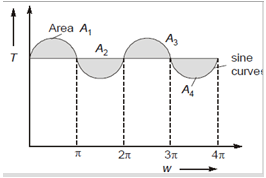Detailed Solution for Practice Test: Mechanical Engineering (ME)- 2 - Question 20 we know,

emax=I ks ωavg2

I = m k2

I = 220.5×kgm2

emax = 220.5×ks×502 = 551250×Ks

Ks=1.5 % + 1.5% = 3%= 0.03

emax=551250×0.03

emax =16537.5 Nm/s

∴ A1=A2=A3=A4=16537.5 Nm/s

Practice Test: Mechanical Engineering (ME)- 2 - Question 21

Newton-Raphson method is used to find the roots of the equation. x3+2x2+3x-1 =0If the initial guess is x0 = 1, then the value of x after 2nd iteration is _____?

Detailed Solution for Practice Test: Mechanical Engineering (ME)- 2 - Question 21 x3+2x2+3x-1 =0

x0 = 1

As per Newton-Raphson Method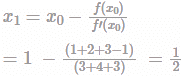Similarly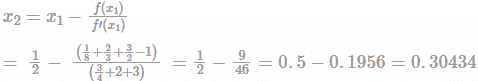Practice Test: Mechanical Engineering (ME)- 2 - Question 22

A thin cylinder of internal diameter 60 mm and wall thickness 2mm is subjected to an internal pressure of 1.25 N/mm2. The cylinder is also subjected to a torque of 60 Nm, the axis of the torque coinciding with that of the cylinder. The minor principal stress is MPa.

Detailed Solution for Practice Test: Mechanical Engineering (ME)- 2 - Question 22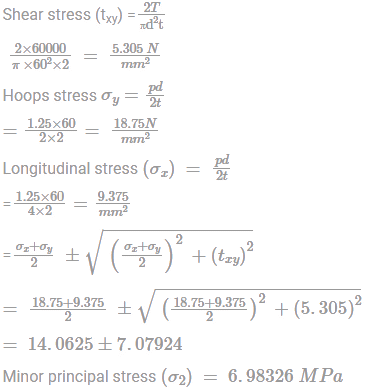Practice Test: Mechanical Engineering (ME)- 2 - Question 23

For the mechanism shown below Find the ratio of angular velocities of the link 2 and link 4.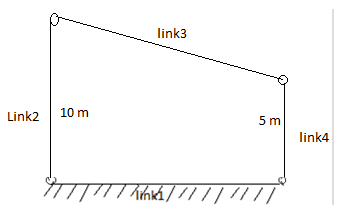Detailed Solution for Practice Test: Mechanical Engineering (ME)- 2 - Question 23 As shown in the above figure the link 2 and link 4 are perpendicular to link 1 so the coupler will go on translational motion as IC23 will on positive infinity.

Under such case the velocity end link 2 = velocity of end link 4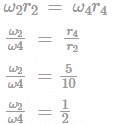Practice Test: Mechanical Engineering (ME)- 2 - Question 24

Consider the following linear programming problem:

Maximize Z =2A+3B, Subject to A+B ≤10, 4A+6B≤30, 2A+B≤17, A,B≥0

What can one say about the solution?

Detailed Solution for Practice Test: Mechanical Engineering (ME)- 2 - Question 24 Z = 2A + 3B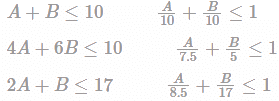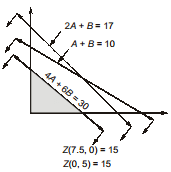Maximum value of objective functions = 15 and it has alternative optimal solutions because of same constraint as objective function.

Practice Test: Mechanical Engineering (ME)- 2 - Question 25

For the differential equation dy/dx + ay = 0 with y(0) = 1, solution is?

Detailed Solution for Practice Test: Mechanical Engineering (ME)- 2 - Question 25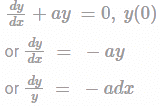Taking integration on both sides, we get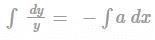or ln y = -ax+c

or y = 1, x = 0 follow initial condition.

ln 1 = -ax 0+c

∴ C = ln 1 = 0

So, ln y = -ax+C

ln y = -ax +0

∴y = e-at .

Practice Test: Mechanical Engineering (ME)- 2 - Question 26

A bullet of weight 1.4g enters a wooden partition block at a velocity of 955 m/s and 0.003s after entering the block. Determine the length penetration of bullet.

Detailed Solution for Practice Test: Mechanical Engineering (ME)- 2 - Question 26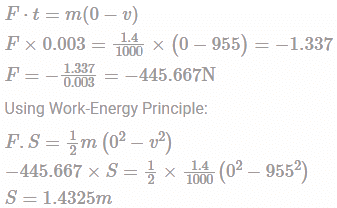Practice Test: Mechanical Engineering (ME)- 2 - Question 27

In a JIT system, demand for a particular product is 2000 units. The safe stock is 15% of authorised inventory. The product spends 0.06 day in handling and 0.04 in processing. How many containers are to be authorised if 1 can contains 10 parts.

Detailed Solution for Practice Test: Mechanical Engineering (ME)- 2 - Question 27 Demand during lead time = 2000 × (0.06 + 0.04) = 200

Safety stock = 0.15 × 200 = 30

No. of containers = (200+30)/10 = 23

Practice Test: Mechanical Engineering (ME)- 2 - Question 28

A steel tube having inner radius 1 cm and outer radius 2.5 cm is covered with asbestos of thickness 3cm. the temperature difference between the outer surface of asbestos and inner surface of steel is 600°C. Ksteel=19w/m2k and Kasbestos= 0.2 w/m2k. Calculate the heat transfer rate per unit length of the pipe in W/m

Detailed Solution for Practice Test: Mechanical Engineering (ME)- 2 - Question 28 r1= 1 cm, r2= 2.5 cm, r3= 2.5+3 = 5.5 cm

Heat transfer rate q is given as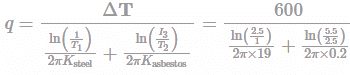= 944.7 W/m

Practice Test: Mechanical Engineering (ME)- 2 - Question 29

The sales of car in a showroom in first, second and third months are 70, 68 and 82 respectively. With the smoothing constant of 0.4, Find the forecast for the next month.

Detailed Solution for Practice Test: Mechanical Engineering (ME)- 2 - Question 29 Ft = Ft-1+ α (Dt-1 - Ft-1)

D1 = 70, D2=68, D3= 82 (given)

Note:- If initial forecasting is not given. you can assume it equal to the demand of that month.

Therefore, F1=70

Now according to formula given above,

F2= F1+α(D1-F1)= 70+0.4(70-70)= 70

F3= F2+α(D2-F2)= 70+0.4(68-70)= 69.2

Therefore, F4= F3+α(D3-F3)= 69.2+0.4(82-69.2)= 74.32

Practice Test: Mechanical Engineering (ME)- 2 - Question 30

Square root of ratio of load required to buckle a column fixed on both ends to a column fixed on one end, free at other end is _____. The geometric length of both columns is same.

Detailed Solution for Practice Test: Mechanical Engineering (ME)- 2 - Question 30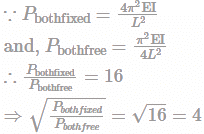Practice Test: Mechanical Engineering (ME)- 2 - Question 31

At point in a body stresses developed are such that σx =140MPa and σy = 60

MPa and the major principle stress is 150 MPa. What is the magnitude of max in-plane shear stress

Detailed Solution for Practice Test: Mechanical Engineering (ME)- 2 - Question 31 Given

σx=140MPaσy=60MPa

Let τmax be the max in plane shear stress.

We know that: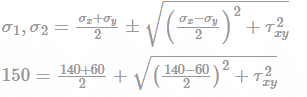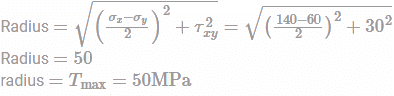Practice Test: Mechanical Engineering (ME)- 2 - Question 32

There is a car of mass m on a platform of mass M. The platform is kept on a smooth ice as shown in fig. If the car starts moving on the platform with a velocity V relative to platform then the velocity of platform recoil relative to ice is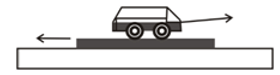Detailed Solution for Practice Test: Mechanical Engineering (ME)- 2 - Question 32 Suppose the velocity of platform recoil and car is w m/s and u m/s both relative to ice respectively. The situation is shown in fig.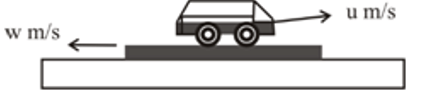Speed of car relative to platform = u+w

And according to question, V = u+w

So, the car velocity relative to ice, u = V – w

Assume the platform and car as a system, there is no external force acting on system i.e., linear momentum of system will be conserved. Initially there is no movement, so Initial linear momentum will be zero.

Initial linear momentum = Final linear momentum

0 = mu-Mw

0 = m (V-w)- Mw

w = mV/(M+m)

Practice Test: Mechanical Engineering (ME)- 2 - Question 33

A bullet of mass m1=20 gm fired horizontally with a velocity of v=200 m/s hits a wooden block of mass m2 =500 gm (take g=9.8m/s2) resting on a horizontal plane as shown in the figure and the bullet remains embedded in the block after the impact. If the coefficient between the surfaces in contact remains constant at 0.3, the distance the block will move before coming to rest is: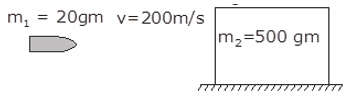Detailed Solution for Practice Test: Mechanical Engineering (ME)- 2 - Question 33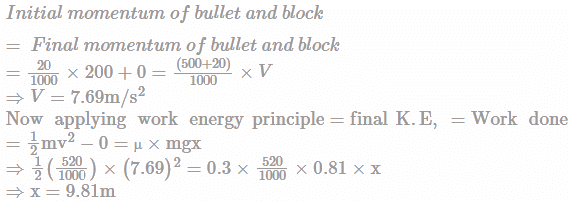Practice Test: Mechanical Engineering (ME)- 2 - Question 34

The height of water level in a tank above the centre of a circular hole 2.5 cm in diameter is 50m. The velocity of water flowing through the hole is (neglect friction between jet and wall)

Detailed Solution for Practice Test: Mechanical Engineering (ME)- 2 - Question 34 Using Bernoulli’s equation, √(2gh(t)), where g is gravity and h(t) is the height of the fluid in the tank at any time.

= √(2x9.81x50) = 31.32 m/s

Note:

The times to empty the same tank for two different starting heights, H1 and H2 is: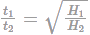So, finally, if H2 = 2H1, as in the problem statement, then the time to empty the tank is not double, but √2 times longer.

Practice Test: Mechanical Engineering (ME)- 2 - Question 35

Two blocks are connected by a light strings as shown in fig. The coefficient of friction at each surface is 0.25 and assumes the pulley is frictionless. The minimum value of m (in kg) for which system remain at rest is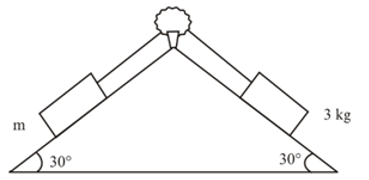Detailed Solution for Practice Test: Mechanical Engineering (ME)- 2 - Question 35 The minimum value of m will be when 3 kg block slides downward. The forces acting on blocks as shown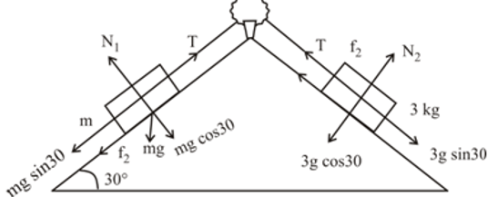At equilibrium

T=mgsin⁡θ+μmgcos⁡θ..........(i)

As pulley is frictionless so, the tension T will be same in whole string,

So,T+3×μgcos⁡θ=3×gsin⁡θ.............(ii)

Putting the values of μ and θ in (i) and (ii) and solved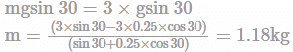*Answer can only contain numeric values
Practice Test: Mechanical Engineering (ME)- 2 - Question 36

The rigid bar AB, attached to two vertical rods as shown in figure, is horizontal before the load P is applied. The vertical movement(mm) of P is _____. Given P = 50 kN.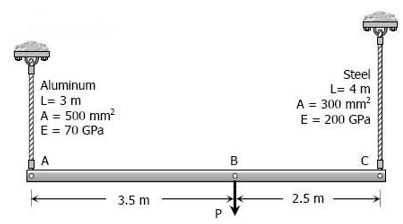Detailed Solution for Practice Test: Mechanical Engineering (ME)- 2 - Question 36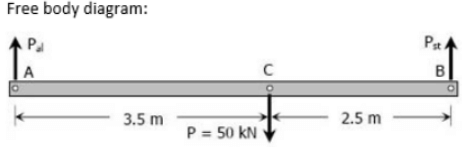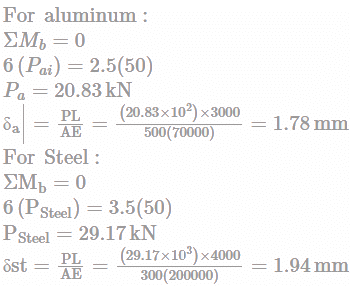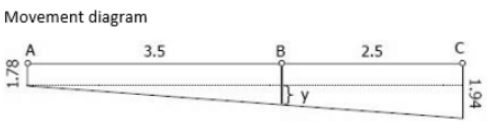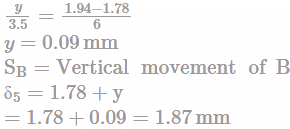Practice Test: Mechanical Engineering (ME)- 2 - Question 37

The solution of differential equation dy/dx = ex-y - x2e-y with y(0)=1 is

Detailed Solution for Practice Test: Mechanical Engineering (ME)- 2 - Question 37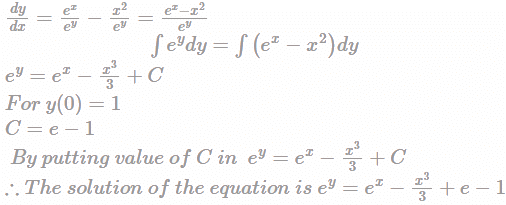Practice Test: Mechanical Engineering (ME)- 2 - Question 38

Match the following :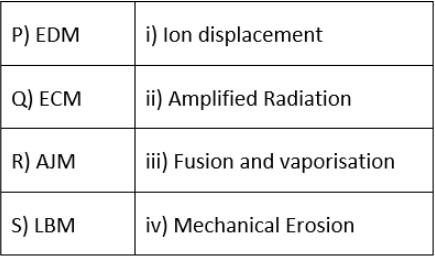Detailed Solution for Practice Test: Mechanical Engineering (ME)- 2 - Question 38 P) EDM - iii) Fusion and vaporization

Electrical discharge machining or EDM, is a non-traditional method in which material is removed from a workpiece using thermal energy.

Q) ECM - i) Ion displacement

ECM is used to machine workpieces through the anodic dissolution of metal.

R) AJM- iv) Mechanical Erosion

The process makes use of an abrasive jet with high velocity, to remove material and provide smooth surface finish to hard metallic workpieces

It works on the principle that when a high energy laser beam strikes the surface of the work piece.

Practice Test: Mechanical Engineering (ME)- 2 - Question 39

A wall of 0.5 m thickness is to be constructed from a material which has an average thermal conductivity of 1.4 W/m-K. The wall is to be insulated with a material having an average thermal conductivity of 0.35 W/m-K so that the heat loss per square metre will not exceed 1450 W. Assuming that the inner and outer surface temperature are 1200 °C and 15 °C respectively. The thickness of insulation required is ____ metre.

Detailed Solution for Practice Test: Mechanical Engineering (ME)- 2 - Question 39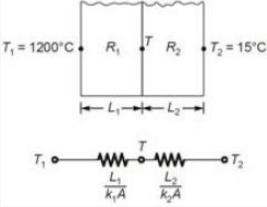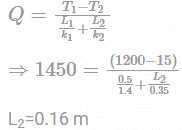Practice Test: Mechanical Engineering (ME)- 2 - Question 40

The blocks shown in the figure has 1 kg. The rear block moves with a speed of 2m/s towards the front block kept at rest. The spring attached to the front block is light and has a spring constant of 50 N/m. The maximum compression of the spring in meters is___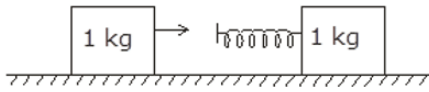Detailed Solution for Practice Test: Mechanical Engineering (ME)- 2 - Question 40 Maximum compression will take place when the blocks move with equal velocity.

Using conservation of linear momentum,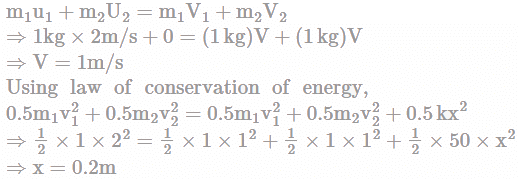Practice Test: Mechanical Engineering (ME)- 2 - Question 41

Steam is condensing in a condenser at 100°C by a fluid which enter at 30°C and leaves at a higher temperature. The NTU of the heat exchanger is 3.2. Find the exit temperature of the fluid used for condensing steam in

Detailed Solution for Practice Test: Mechanical Engineering (ME)- 2 - Question 41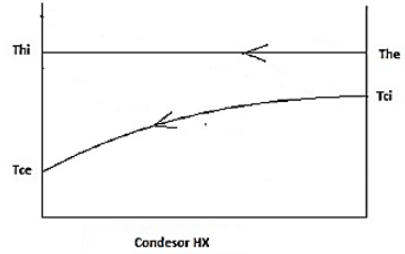Thi=The=100°C

Tci=30°C (The flow direction of fluid doesn’t have any effect in phase changing fluid HX)

ε=1-e-NTU

ε=1-e-3.2

ε=0.959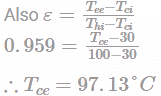Practice Test: Mechanical Engineering (ME)- 2 - Question 42

A casting of size 150 × 150 × 30 mm is not a side riser. The surface area of casting is 63000 mm2, having velocity of 2.14 m/s and volume flow rate as 0.245 m3/s. What would be the modulus of casting.

Detailed Solution for Practice Test: Mechanical Engineering (ME)- 2 - Question 42 The casting does not have a side riser.

Therefore, Modulus of casting = Volume / Surface area

Volume of casting = 150 × 150 × 30 = 675000 mm3

Modulus of casting = 675000/63000 = 10.71

Note: Exothermic Riser Modulus is defined as the ratio of casting volume divided by the casting surface area. Normally, it is sufficient to calculate the modulus at the thickest section of the casting.

Practice Test: Mechanical Engineering (ME)- 2 - Question 43

In a cooling tower, the DBT and WBT of air is 28°C and 23°C respectively while the temperature of water at inlet and outlet states is 32°C and 24°C respectively. The approach and range (in °C ) in cooling tower respectively is

Detailed Solution for Practice Test: Mechanical Engineering (ME)- 2 - Question 43 Approach in cooling tower = Water temperature at the outlet of cooling tower- WBT of entering air = 24 - 23

Approach = 1°C

Range in cooling tower = Water temperature at the inlet states of cooling tower - water temperature at the exit states of cooling tower = 32 – 24

Range = 8°C

Practice Test: Mechanical Engineering (ME)- 2 - Question 44

In a spring mass damper system (k=20N/m; m=2kg), The value of damped frequency (rad/sec) if the damper offers a resistance of 2N force when it vibrates with a constant velocity of 40cm/sec is:

Detailed Solution for Practice Test: Mechanical Engineering (ME)- 2 - Question 44 Damping force F=Cx

x=40cmsec 0.4m/sec and F=2N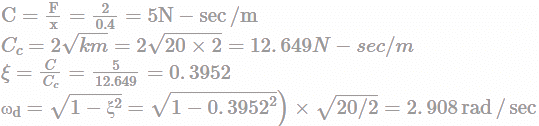Practice Test: Mechanical Engineering (ME)- 2 - Question 45

A cantilever beam with the bending moment M at the free end is shown in the diagram. The radius of Mohr’s circle of the element P is 7 MPa.What is the load (kN-m) applied at the free end?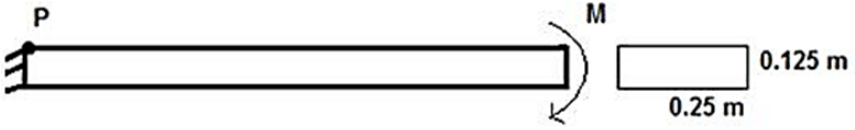Detailed Solution for Practice Test: Mechanical Engineering (ME)- 2 - Question 45 For Point p radius of Mohr's circle is equal half of the stress at the P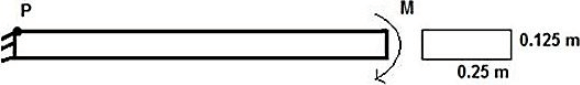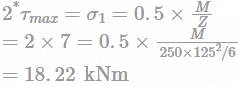Practice Test: Mechanical Engineering (ME)- 2 - Question 46

A dealer for washing machines forecasts the demand at the rate of 600 units per month, for the next four months. The actual demand is found to be 500, 680, 800 and 900 units. The mean absolute deviation (MAD) and the BIAS are found to be

Practice Test: Mechanical Engineering (ME)- 2 - Question 47

Find the value of number of standard normal variate for the critical path, If the project completion time is 19 days.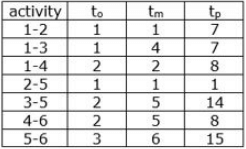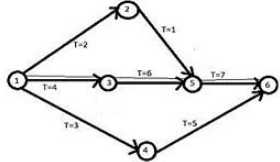Detailed Solution for Practice Test: Mechanical Engineering (ME)- 2 - Question 47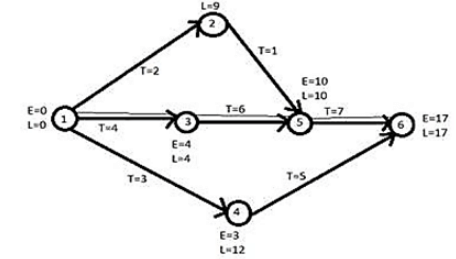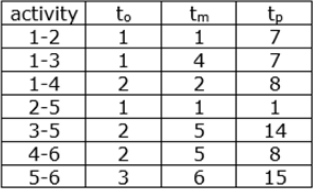σ13=(7-1)/6=1

σ35=(14-2)/6=2

σ56=(15-3)/6=2

σcr=(12+22+22)(1/2)=3

Z(standard normal variate)

= (T - Tcp)/(σcr) = (19 - 17)/3 = 0.67

Practice Test: Mechanical Engineering (ME)- 2 - Question 48

Blind holes of 20 mm diameter, 100 mm deep are being drilled in steel block. Drilling spindle speed is 600 rpm, feed is 0.2 mm/rev, point angle of drill is 135 °. During this operation, the drill wears out after producing 300 holes. Taylor’s tool life equation is of the form VT0.3 = C, where V = cutting speed in m/min and T = tool life in minutes Taylor’s constant C will be _____.

Detailed Solution for Practice Test: Mechanical Engineering (ME)- 2 - Question 48 L=L1+L2+L3+L4

L1=Depth of the hole=100mm

L2=Approach length =0mm

L3=Length of tip=0.5D/tanθ =0.5×20/tan67.5°=4.14

(where,2θ=point angle or lip angle)

L4=Over travel=0mm

⇒L=L1+L3=104.142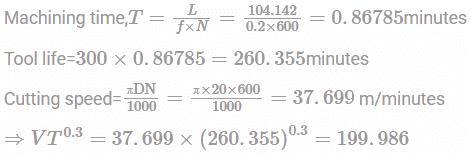Practice Test: Mechanical Engineering (ME)- 2 - Question 49

In a single heater regenerative cycle (as shown in fig.), the 1 kg of steam enters the turbine at 25 bar, 400°C and the exhaust pressure is 0.1 bar. The feed water heater of direct contact type operates at 5 bar.

The values of enthalpies at different states are shown in table: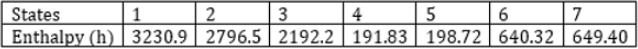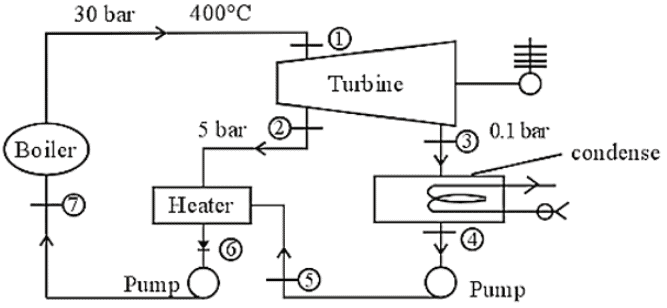If the entropy at states 1, 4 and 6 are 6.9212 KJ/kgK, O.6493 KJ/kgK and 1.867 KJ/kgK respectively, then the increase in mean temperature of heat addition (in∘C) after heat regeneration is

Detailed Solution for Practice Test: Mechanical Engineering (ME)- 2 - Question 49 The mean temperature with regeneration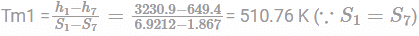Tm1 = 237.76°C

Mean temperature of heat addition without regeneration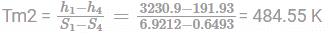Tm2 = 211.5°C

The increase in mean temperature of heat addition

Tm = 237.76-211.5 = 26.26°C

Practice Test: Mechanical Engineering (ME)- 2 - Question 50

A rigid beam AB is supported as shown in figure. The rod AM is of steel, with a cross-sectional area of 10 cm2, the rod BN is of wood with a cross-sectional area of 100 cm2, the rod CD is of copper, and has cross-sectional area of 30 cm2. The displacement of the point of application of the load is [Take Es=200 G Pa, Ewood=10GPa]  Young's modulus of copper =130 GPA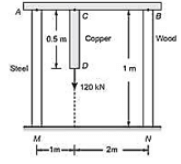Detailed Solution for Practice Test: Mechanical Engineering (ME)- 2 - Question 50

Ps+Pw=120kN

∑Ms=0

⇒3Ps=120×2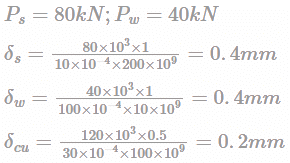Both steel and wood plate get compressed by same length hence

δD= 0.4+0.2= 0.6mm.

Practice Test: Mechanical Engineering (ME)- 2 - Question 51

A house that is losing heat at a rate of 60,000 kJ/hr when the outside temperature drops to 4 °C, is to be heated by electric resistance heaters. If the house is to be maintained at 24 °C at all times, then the irreversibility (in kW) of this process is

Detailed Solution for Practice Test: Mechanical Engineering (ME)- 2 - Question 51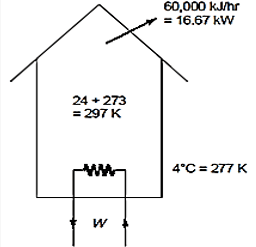The reversible work is the minimum work required to accomplish this process, and the irreversibility is the difference between the reversible work and the actual electrical work consumed.

Actual power input

Woct'in = Qout = QH=60000 kj/h

=16.67 kW

COP of a reversible heat pump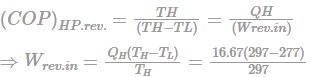=1.1225 kW

I=Woct.in - Wrev.in

=16.67-1.1225

=15.55 kW

Practice Test: Mechanical Engineering (ME)- 2 - Question 52

If demand of a certain item doubles then the change in Economic Order Quantity is given as:

EOQnew = nEOQold

What will be the value of n?

Detailed Solution for Practice Test: Mechanical Engineering (ME)- 2 - Question 52 The relationship between EOQ and Demand (U) is given by,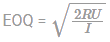Where R and I are ordering and inventory cost of 1 item,

This shows that: EOQ ∝ √U

21/2

Practice Test: Mechanical Engineering (ME)- 2 - Question 53

A random variable X has probability density function f(x) as given below.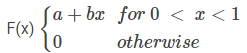If the expected value E[X] = ⅔, then Pr [X < 0.5]="" />

Detailed Solution for Practice Test: Mechanical Engineering (ME)- 2 - Question 53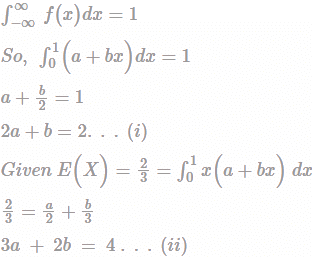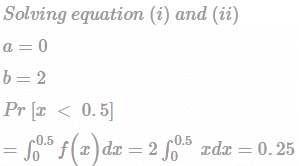Practice Test: Mechanical Engineering (ME)- 2 - Question 54

If s is the stroke of follower, θo and θr being angular displacement of cam during outstroke and return stroke and ω is angular velocity of cam, then acceleration of the follower during return stroke will be:

Detailed Solution for Practice Test: Mechanical Engineering (ME)- 2 - Question 54 If s is the stroke of follower, θo and θr being angular displacement of cam during outstroke and return stroke and ω is angular velocity of cam, then time required for follower outstroke will be to = θ0 / ώ while time required for follower return stroke = tr = θr / ώ. Now the average velocity of follower will be = s/t.

Hence the average velocity of follower during outstroke = s/2 / to/2

= s/ to

= vomin + vomax/2

As vomin = 0, so

vomax = 2s / to

= 2ώs / θ0

Average velocity of follower during return stroke = s/2 / tr/2

= s/tr

= vrmin + vrmax/2

As vrmin = 0, so

vrmax = 2s / tr

= 2ώs / θr which is max velocity during return stroke.

Acceleration of the follower during outstroke = a0 = vomax / t0/2 = 4ώ2s / θ02

Acceleration of the follower during return stroke = ar = vrmax / t0/2 = 4ώ2s / θr2

ar = 4ώ2s / θr2

Practice Test: Mechanical Engineering (ME)- 2 - Question 55

Two large parallel plates having temperatures T1 = 1500K and T2 = 500K are kept in such a way that radiation leaving from each surface is completely reached the 2nd surface. Emissivity values for plates are 0.5 and 0.25 respectively. Then heat transfer between the plates(in kW/m2)

Detailed Solution for Practice Test: Mechanical Engineering (ME)- 2 - Question 55 Given,

Two large parallel plates,

temperatures T1 = 1500K

temperatures T2 = 500K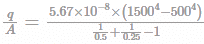= 56.7 kW/m2
Practice Test: Mechanical Engineering (ME)- 2 - Question 56

An increase in pressure of 500 kPa and decrease in volume by 0.02%, and Poisson ratio is 0.3. What is value of young modulus of elasticity?

Detailed Solution for Practice Test: Mechanical Engineering (ME)- 2 - Question 56 Bulk modulus k = Δp/(-Δv/v)

K = 500 × 103/ (0.0002)

K = 2.5 × 109 Pa

µ = 0.3 (given)

as we know the relation

E = 3K (1- 2µ)

E = 3 × 2.5 ×109 (1- 2×0.3)

E = 3 × 109 Pa

Practice Test: Mechanical Engineering (ME)- 2 - Question 57

Two very large parallel plane with emissivity’s 0.3 and 0.8 exchange radiative energy. The percentage reduction in radiative energy transfer when a polished aluminium radiation shield (∈=0.05) is placed between them is __________%.

Detailed Solution for Practice Test: Mechanical Engineering (ME)- 2 - Question 57 The radiation heat transfer rate without shield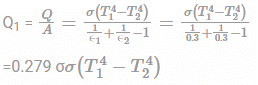Rate of radiation heat transfer with one shield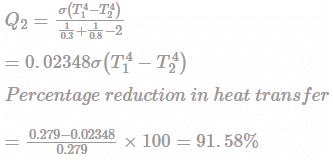Practice Test: Mechanical Engineering (ME)- 2 - Question 58

A radial load f1 = 5.6 kN acts for two hours on a rolling bearing and then reduces to f2 = 3.3 kN for one hour. The cycle then repeats itself. the shaft rotates at 300 rev/ min. What will be mean cubic load (fm), which should be used in rating the bearing for a life it 12,000 hour

Detailed Solution for Practice Test: Mechanical Engineering (ME)- 2 - Question 58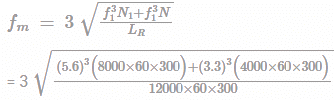= 2.905 kN

Practice Test: Mechanical Engineering (ME)- 2 - Question 59

The weight of two people standing on a see saw at points A and B are measures 300N and 200N respectively. If the see saw is resting on its supports and weighs 500N, find the upward force P?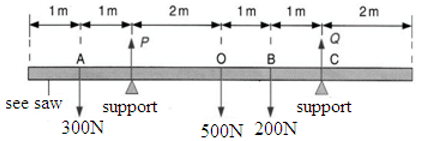Detailed Solution for Practice Test: Mechanical Engineering (ME)- 2 - Question 59 From the figure, sum of forces in a direction is sum of forces in opposite direction, so

P + Q

= 300N + 500N + 200N

= 1000N

Now taking moments at point C due to Q, so clockwise moment will be:

= P x 4m

Also, anticlockwise moment will be:

= (300N x 5m) + (500N x 2m) + (200N x 1m)

= 2700Nm

Further, Clockwise Moment = Anticlockwise Moment

So: P x 4m = 2700Nm

P = 2700Nm/4

P = 675N

Practice Test: Mechanical Engineering (ME)- 2 - Question 60

There is a vertical engine, having piston at the top and crank at the bottom, running at 2400 rpm. The length of crank and connectivity rod is 50 mm and 300 mm respectively. The diameter of the pistons is 100 mm and the mass of the reciprocating parts is 1.5 kg. The crank has turned 30 from the top dead centre during the expansion stoke and the gas pressure is 600 kN/m2. The net force on the piston is _____ N.

Detailed Solution for Practice Test: Mechanical Engineering (ME)- 2 - Question 60 Given

Length of crank, r = 50 mm = 0.05 m

Length of connecting rod, l = 300 mm = 0.3 m

N = 2400 rpm

Gas pressure, P = 600 kN/m2

Mass of reciprocating parts, m = 1.5 kg

Diameter of piston, d = 0.1 m

θ = 30o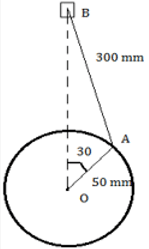Now,

n = l/r = 0.3/0.05 = 6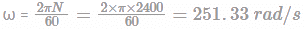=4712.39 N

Inertia force, Fb = mrω2 (cos θ+ (Cos2θ/n))

Net force on the piston, F

F = Fp – Fb + mg = 4712.39 – 4497.59 + 1.5 x 9.81 =229.52 N ≈229 N

Practice Test: Mechanical Engineering (ME)- 2 - Question 61

Let x and y be integers satisfying the following equations

2x2+y2 = 34

x + 2y = 11

The value of (x+y) is ________.

Detailed Solution for Practice Test: Mechanical Engineering (ME)- 2 - Question 61 2x2 + y2 = 34 ......(1)

x + 2y = 11 .......(2)

From equation (2)

x = 11-2y now put the value of x in equation (1)....

2(11-2y)2 + y2 = 34

2 (121 + 4y2 + 44y) + y2 = 34

242 + 8 y2 -88y + y2 = 34

9 y2 - 88y + 208 = 0

On solving using Shridhar Acharya’s method we got

y = 4 & 52/9

Since, x +2y = 11

x = 11 - 8 = 3

So, x + y = 7

Practice Test: Mechanical Engineering (ME)- 2 - Question 62

To compress a helical spring that is already compressed, where

Lo = 5.0 cm = 0.05 m

Li = 4.5 cm = 0.045 m

Lf = 3.5 cm = 0.035 m

k = 18,000 N/m

The work done will be:

Detailed Solution for Practice Test: Mechanical Engineering (ME)- 2 - Question 62 Step 1: using eq.: x1 = Li – Lo, x2 = Lf – Lo; Calculate the displacements (x1) and (x2) as

x1 = Li − Lo = (0.045 m) − (0.05 m)

= −0.005 m

x2 = Lf − Lo = (0.035 m) − (0.05 m)

= −0.015 m

Step 2: Substitute the displacements (x1) and (x2) found in step 1 in Equation to give the work done as

Work1→2 = (1/2) k(x21 − x22)

= 1/2(18,000 N/m) × ((−0.005 m) 2 − (−0.015 m) 2)

= 1/2(18,000 N/m) × ((0.000025 − 0.000225) m2)

= 1/2(18,000 N/m)x (−0.0002 m2)

= −1.8 N · m = −180 N · cm

The negative sign on the work done means work was done on the spring.

Practice Test: Mechanical Engineering (ME)- 2 - Question 63

A longitudinal copper tin (k=380 W/m-K) 600 mm long and 5 mm diameter is expose of air stream at 200C. The convective heat transfer coefficient is 20 W/m2-K. If the tin base temperature is 1500C, then efficiency of the tin __________%.

Detailed Solution for Practice Test: Mechanical Engineering (ME)- 2 - Question 63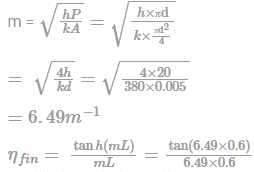= 25.66%

Practice Test: Mechanical Engineering (ME)- 2 - Question 64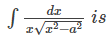Detailed Solution for Practice Test: Mechanical Engineering (ME)- 2 - Question 64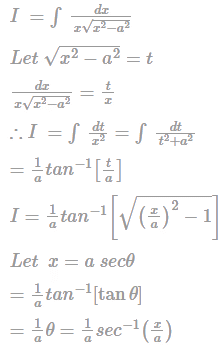Practice Test: Mechanical Engineering (ME)- 2 - Question 65

A piston-cylinder device contains 0.05 m3 of gas initially at 180 kPa. At this stage, a linear spring that has a spring constant of 150 kN/m is just touching the piston but exerting no force on it. Now the heat is transferred to the gas, causing the piston to rise and to compress the spring until the volume inside the cylinder doubles. If the cross-sectional area of the piston is 0.25 m2, then the total work done (in kJ) by the gas will be

Detailed Solution for Practice Test: Mechanical Engineering (ME)- 2 - Question 65 Assumption: The expansion process is quasi-equilibrium and spring is linear in the range of interest.

V2 = 2V = 2 × 0.05 = 0.1 m3

Displacement of the piston (and of the spring)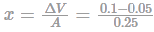=0.5 m

Additional pressure applied by the spring on the gas at final state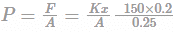=120 kPa

Without the spring, the pressure of the gas would remain constant at 180 kPa while the piston is rising. But under the effect of the spring, the pressure rises linearly from 180 kPa to 180 + 120 = 300 kPa at the final state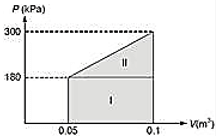Total work done = Work done against the atmosphere + Work done against the spring

= Area I + Area II

= (300 + 180)/2 (0.1 - 0.05).

Alternate:

Total work done by the gas = Work done in expansion + Work saved in the form of potential energy of spring =180(0.1-0.05)+(½)×150×0.22=12 kJ

## GATE Mechanical (ME) 2023 Mock Test Series

26 docs|239 tests
 Use Code STAYHOME200 and get INR 200 additional OFF Use Coupon Code
Information about Practice Test: Mechanical Engineering (ME)- 2 Page
In this test you can find the Exam questions for Practice Test: Mechanical Engineering (ME)- 2 solved & explained in the simplest way possible. Besides giving Questions and answers for Practice Test: Mechanical Engineering (ME)- 2, EduRev gives you an ample number of Online tests for practice

## GATE Mechanical (ME) 2023 Mock Test Series

26 docs|239 tests